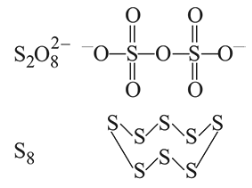# The number of bonds between sulphur and oxygen atoms inQuestion:

The number of bonds between sulphur and oxygen atoms in $\mathrm{S}_{2} \mathrm{O}_{8}^{2-}$ and the number of bonds between sulphur and sulphur atoms in rhombic sulphur, respectively, are:

1. 4 and 6

2. 8 and 8

3.  8 and 6

4. 4 and 8

Correct Option: , 2

Solution: